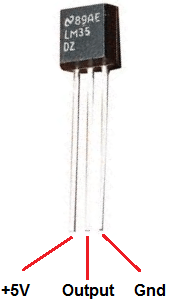# STM32 Nucleo and LM35 temperature sensor

210

In this example we will connect an LM35 temperature sensor to our Nucleo board and we will be using the MBed compiler

The LM35 series are precision integrated-circuit temperature sensors, whose output voltage is linearly proportional to the Celsius (Centigrade) temperature. The LM35 thus has an advantage over linear temperature sensors calibrated in Kelvin, as the user is not required to subtract a large constant voltage from its output to obtain convenient Centigrade scaling. The LM35 does not require any external calibration or trimming to provide typical accuracies of ±1/4°C at room temperature and ±3/4°C over a full -55 to +150°C  temperature range

Here is a picture of the pins, its important to get these correct or you can damage the sensorSchematics and Connection

Very simple to connect

Vcc is 3v3
Gnd is any Gnd and output goes to Nucleo A0, you can see this below

Code

This example is for the mbed online compiler – https://os.mbed.com/compiler/

 Source code```#include "mbed.h"

Serial pc(SERIAL_TX, SERIAL_RX); //Create an object of Serial Class

int main()
{
float tempC,tempF,a,avg;
int i;

while(1)
{

avg=0;
for(i=0;i<10;i++)
{
wait(.02);
}
for(i=0;i<10;i++)
{
avg=avg+(a[i]/10);
}

tempC=(avg*3.685503686*100);
tempF=(9.0*tempC)/5.0 + 32.0;
pc.printf("temperature = %.0f ^C\r\n", tempC);

wait(1);
}
}```

Output

Using a terminal program you should see something like this

temperature = 21 ^C
temperature = 21 ^C
temperature = 21 ^C
temperature = 21 ^C
temperature = 23 ^C
temperature = 24 ^C
temperature = 25 ^C
temperature = 25 ^C
temperature = 26 ^C
temperature = 26 ^C
temperature = 26 ^C